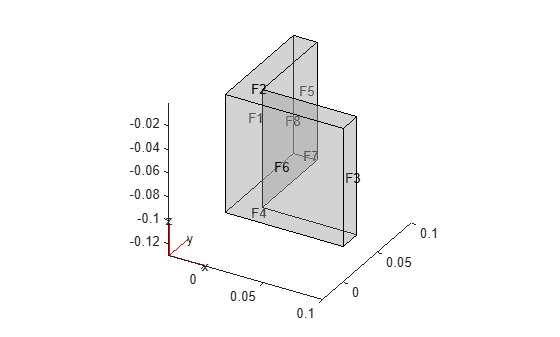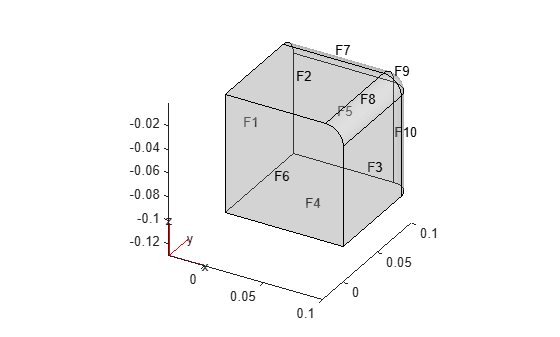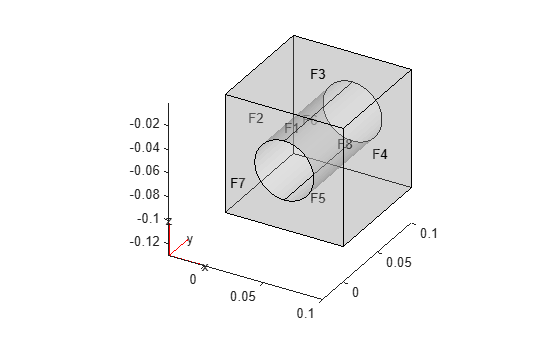# STEP File Import

This example shows how to import a geometry from a STEP file and then plot the geometry. After importing, view the geometry using the `pdegplot` function.

Import and view the geometry examples from the STEP files included with Partial Differential Equation Toolbox™. To see the face IDs, set the `FaceLabels` name-value argument to "`on"`. To see the labels on all faces of the geometry, set the transparency to 0.3.

```figure gm = fegeometry("AngleBlock.step"); pdegplot(gm,FaceLabels="on",FaceAlpha=0.3)``````figure gm = fegeometry("AngleBlockBlendR10.step"); pdegplot(gm,FaceLabels="on",FaceAlpha=0.3)``````figure gm = fegeometry("BlockBlendR15.step"); pdegplot(gm,FaceLabels="on",FaceAlpha=0.3)``````figure gm = fegeometry("BlockWithHole.step"); pdegplot(gm,FaceLabels="on",FaceAlpha=0.3)```